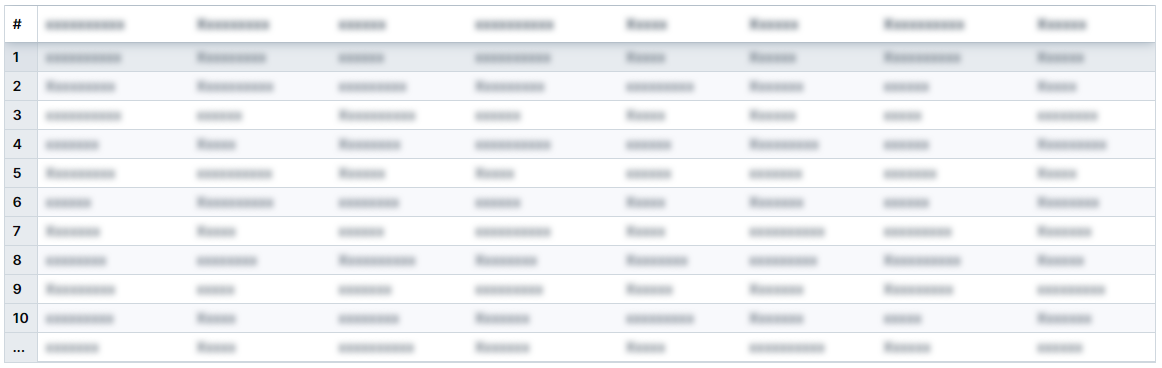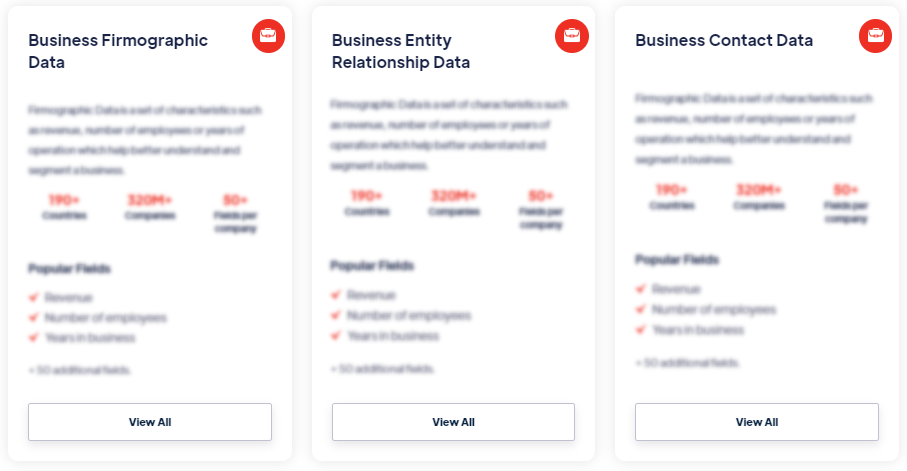# Products

An ROC curve is a plot that visualizes the performance of a binary classification model. It shows how well the model can distinguish between the positive and negative classes by varying the classification threshold. The x-axis represents the false positive rate (FPR), and the y-axis represents the true positive rate (TPR). Read more

#### Our Data Integrations### ROC Curve### Browse the Data Marketplace1. What is an ROC Curve?
An ROC curve is a plot that visualizes the performance of a binary classification model. It shows how well the model can distinguish between the positive and negative classes by varying the classification threshold. The x-axis represents the false positive rate (FPR), and the y-axis represents the true positive rate (TPR).

2. How is an ROC Curve Constructed?
To construct an ROC curve, the model's predictions and true labels are used. The classification threshold is adjusted, and for each threshold value, the TPR and FPR are calculated. The TPR is the proportion of true positives correctly classified, and the FPR is the proportion of false positives incorrectly classified. These values are plotted to create the ROC curve.

3. What Does the ROC Curve Show?
The ROC curve illustrates the model's performance across various threshold values. Each point on the curve represents a different classification threshold, and the curve itself shows the relationship between TPR and FPR. A better-performing model will have an ROC curve that is closer to the top-left corner of the plot, indicating a higher TPR and lower FPR for a range of thresholds.

4. How is the ROC Curve Interpreted?
The ROC curve allows for the evaluation of the model's trade-off between true positive rate and false positive rate. A point on the curve corresponds to a specific classification threshold, and the choice of threshold determines the balance between sensitivity and specificity. The area under the ROC curve (AUC) is a common metric used to summarize the overall performance of the model. A higher AUC indicates better discrimination ability.

5. What are the Benefits of ROC Curves?
ROC curves provide a comprehensive view of a model's performance, allowing for comparison across different classification thresholds. They are particularly useful when the class distribution is imbalanced or when the costs of false positives and false negatives differ. The AUC provides a single metric to quantify the model's discrimination ability, making it easier to compare different models or variations of the same model.

6. Are There Limitations to Using ROC Curves?
While ROC curves are widely used, it's important to consider the specific context and requirements of the classification problem. ROC curves do not provide insights into the overall accuracy or the optimal classification threshold for a specific application. Other evaluation metrics, such as precision, recall, and F1 score, may be more appropriate depending on the specific use case.

7. How Can ROC Curves Aid Model Evaluation?
ROC curves are valuable for assessing and comparing the performance of different classification models. They can help in selecting the best model based on the desired balance between TPR and FPR. Additionally, ROC curves are useful for identifying the optimal threshold that maximizes the model's performance for a specific application. They also provide insights into the model's sensitivity to classification errors and its ability to distinguish between the positive and negative classes.